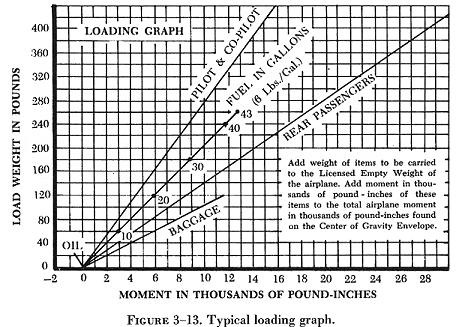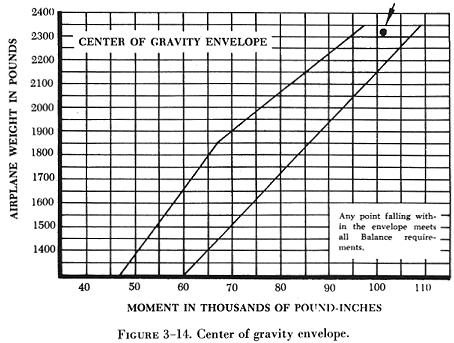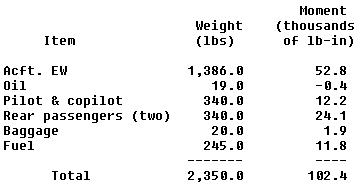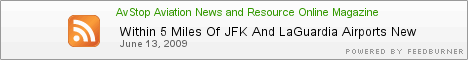LOADING GRAPHS AND CG ENVELOPES The weight and balance computation system, commonly called the loading graph and CG envelope system, is an excellent and rapid method for determining the CG location for various loading arrangements. This method can be applied to any make and model of aircraft. Aircraft manufacturers using this method of weight and balance computation prepare graphs similar to those shown in figure 3-13 and 3-14 for each make and model aircraft at the time of original certification. The graphs become a permanent part of the aircraft records. Along with the graphs are the data for the empty weight arm and moment (index number) for that particular make and model aircraft. The loading graph illustrated in figure 3-13 is used to determine the index number of any item or weight that may be involved in loading the aircraft.

To use this graph, find the point on the vertical scale that represents the known weight. Project a horizontal line to the point where it intersects the proper diagonal weight line (i.e., pilot, copilot, baggage, etc.). From the point of intersection, read straight downward to the horizontal scale to find the moment or index number.After the moment for each item of weight has been determined, all weights are added and all moments are added. With knowledge of the total weight and moment, project a line from the respective point on the CG envelope shown in figure 3-14, and place a point at the intersection of the two lines. If the point is within the diagonal lines, the loading arrangement meets all balance requirements. The following is an actual weight and balance computation using the graphs in figure 3-13 and figure 3-14. For this example, assume that the aircraft has an empty weight of 1,386.0 pounds and a moment of 52,772.0 pound-inches. The index number for the empty weight of the aircraft is developed by dividing the empty weight moment by 1,000. This gives an index number of 52.8 for the airplane's empty weight moment. Load the aircraft to determine whether the CG will fall within the diagonal lines of figure 3-14. Arrange item weights and index numbers in an orderly form to facilitate adding.The total airplane weight in pounds is 2,350.0, and the moment is 102.4. Locate this point (2,350 @ 102.4) on the CG envelope illustrated in figure 3-14. Since the point falls within the diagonal lines, the loading arrangement meets all weight and balance requirements. ©AvStop Online Magazine                                                                   General Handbook